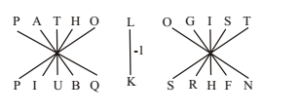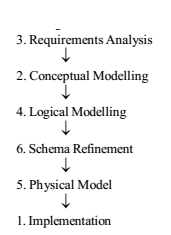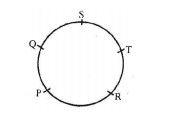# RRB ALP 2018 Practice Test Papers | Reasoning Questions (Day-30)

Dear Aspirants, Here we have given the Important RRB ALP & Technicians Exam 2018 Practice Test Papers. Candidates those who are preparing for RRB ALP 2018 can practice these Reasoning Questions to get more confidence to Crack RRB 2018 Examination.

[WpProQuiz 1979]

Click “Start Quiz” to attend these Questions and view Solutions

1. Three of the following four are alike in a certain way and so form a group. Which is the one that does not belong to that group?

(a) Crow

(b) Vulture

(c) Bat

(d) Ostrich

1. Three of the following four are alike in a certain way and so form a group. Which is the one that does not belong to that group?

(a) Food: Hunger

(b) Water: Thirst

(c) Air: Suffocation

(d) Talent: Education

1. In the following number series a wrong number is given. Find out the wrong number. 3 10 35 172 885 5346 37471

(a) 10

(b) 5346

(c) 885

(d) 35

1. In the following number series a wrong number is given. Find out the wrong number. 318 158 76 38 18 8 3

(a) 38

(b) 18

(c) 158

(d) 76

1. In a certain code PATHOLOGIST is written as PIUBQKSRHFN. How is CONTROVERSY written in that code?

(a) SUOPDNXRQDU

(b) SUOPDNZTSFW

(c) QSMNBPXRQDU

(d) QSMNBPZTSFW

1. Which one of the given responses would be a meaningful order of the following?

1. Implementation 2. Conceptual Modelling 3. Requirements Analysis 4. Logical Modelling 5. Physical Model 6. Schema Refinement

(a) 1, 3, 2, 6, 5, 4

(b) 3, 2, 5, 4, 6, 1

(c) 3, 2, 1, 4, 6, 5

(d) 3, 2, 4, 6, 5, 1

1. P, Q, R, S and T are sitting around a circular table. R is to the right of P and is second to the left of S. T is not between P and S. Who is second to the left of R?

(a) S

(b) Q

(c) T

1. Mohan and Suresh study in the same class. Mohan has secured more marks than Suresh in the terminal examination. Suresh’s rank is seventh from top among all the students in the class. Which of the following is definitely true?

(a) Mohan stood first in the terminal examination.

(b) There is at least one student between Mohan and Suresh in the rank list.

(c) There are at the most five students between Mohan and Suresh in the rank list.

(d) Suresh is five ranks lower than Mohan in the rank list.

1. If 5 + 7 = 21 and 9 + 4 = 31, what is 7 + 9 =?

(a) 41

(b) 51

(c) 61

(d) 71

1. From the given alternatives select the word which cannot be formed using the letters of the given word.

INVESTIGATION

(a) INSTIGATION

(b) GESTATION

(c) VEST

(d) STIGMA

Except it others are birds whereas bat is a mammal

Lack of first one causes second one.

Series is × 2 + 22, × 3 + 32, × 4 + 42

The series is ÷ 2 – 1 in each term.Five letters of the word PATHOLOGIST are reversed first and then coded as one place forward. Similarly, the last five letters of the word are reversed then coded as one place backward. Middle letter is coded as one place backward. Hence, CONTROVERSY will be written as SUOPDNXRQDU.

1. Answer: (d)2. Answer: (b)If, 5 + 7 = 12

=> 21 9 + 4 = 13

=> 31

Then, 7 + 9 = 16 => 61

STIGMA cannot be formed using word ‘INVESTIGATION’ because letter M is not in the given reference word.

RRB ALP 2018 Practice Test Papers | Reasoning Questions (Day-1)

RRB ALP 2018 Practice Test Papers | Reasoning Questions (Day-2)

RRB ALP 2018 Practice Test Papers | Reasoning Questions (Day-3)

RRB ALP 2018 Practice Test Papers | Reasoning Questions (Day-4)

RRB ALP 2018 Practice Test Papers | Reasoning Questions (Day-5)

RRB ALP 2018 Practice Test Papers | Reasoning Questions (Day-6)

RRB ALP 2018 Practice Test Papers | Reasoning Questions (Day-7)

RRB ALP 2018 Practice Test Papers | Reasoning Questions (Day-8)

RRB ALP 2018 Practice Test Papers | Reasoning Questions (Day-9)

RRB ALP 2018 Practice Test Papers | Reasoning Questions (Day-10)

RRB ALP 2018 Practice Test Papers | Reasoning Questions (Day-11)

RRB ALP 2018 Practice Test Papers | Reasoning Questions (Day-12)

RRB ALP 2018 Practice Test Papers | Reasoning Questions (Day-13)

RRB ALP 2018 Practice Test Papers | Reasoning Questions (Day-14)

RRB ALP 2018 Practice Test Papers | Reasoning Questions (Day-15)

RRB ALP 2018 Practice Test Papers | Reasoning Questions (Day-16)

RRB ALP 2018 Practice Test Papers | Reasoning Questions (Day-17)

RRB ALP 2018 Practice Test Papers | Reasoning Questions (Day-18)

RRB ALP 2018 Practice Test Papers | Reasoning Questions (Day-19)

RRB ALP 2018 Practice Test Papers | Reasoning Questions (Day-20)

RRB ALP 2018 Practice Test Papers | Reasoning Questions (Day-21)

RRB ALP 2018 Practice Test Papers | Reasoning Questions (Day-22)

RRB ALP 2018 Practice Test Papers | Reasoning Questions (Day-23)

RRB ALP 2018 Practice Test Papers | Reasoning Questions (Day-24)

RRB ALP 2018 Practice Test Papers | Reasoning Questions (Day-25)

RRB ALP 2018 Practice Test Papers | Reasoning Questions (Day-26)

RRB ALP 2018 Practice Test Papers | Reasoning Questions (Day-27)

RRB ALP 2018 Practice Test Papers | Reasoning Questions (Day-28)

RRB ALP 2018 Practice Test Papers | Reasoning Questions (Day-29)